# Watershed Delineation from a Digital Elevation Model

 Date 28.05.2018 Size 116.51 Kb.
GIS in Water Resources

Fall 2013

Homework #2

1. Watershed Delineation from a Digital Elevation Model

Following is a grid of elevations in a 30 m digital elevation model.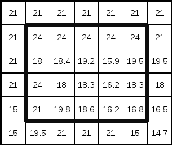1. On the above grid, determine which grid cells are pits and indicate the elevation to which they need to be raised to fill them.

1. For the inner block of 4 x 5 grid cells indicated by the box determine the D8 flow direction and indicate this using an arrow on the diagram below. Use pit filled elevation values.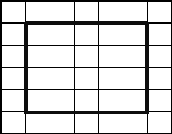Note. Flow directions are not evaluated along the edges because they may depend on data outside the domain. Indicate any grid cells where there is ambiguity and flow direction needs to be arbitrarily chosen. Comment on how it may be good for the computer to evaluate flow direction in these cases.

1. Calculate the D8 slope for the two cells indicated below. Use pit filled elevation values.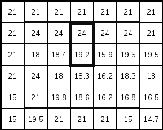1. Calculate the flow accumulation for all grid cells in the inner 4 x 5 block indicated by the bold box. Write your answers (reported in terms of the number of grid cells flowing into each grid cell) in the diagram below. On this diagram also indicate the watershed draining to the grid cell labeled A by drawing a line around it.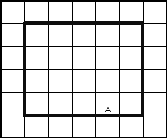1. Determine a stream network using a flow accumulation threshold of 3 grid cells and indicate the grid cells that comprise the stream network. Identify each segment of your stream network using a unique number in the diagram below. You should only fill in stream segment numbers for grid cells on the stream network.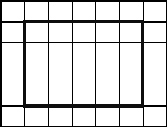1. Determine the grid cells belonging to the catchment draining to each stream segment and fill in numbers in the diagram below indicating the catchment grid.1. Prepare a table giving the length of each stream segment and the drainage area of the corresponding catchment. Also indicate the total area and total length of the stream network draining to grid cell A. Report the drainage density for this stream network. Drainage density is Length/Area.

1. Slope

The following is a grid of elevations in meters in a digital elevation model with 50 m cell size.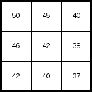(a) Determine the Hydrologic Slope of the center cell with elevation 42

(b) What D8 Flow Direction encoding does this cell have using the ESRI direction encoding?

(c) Use the ArcGIS slope method to determine the x and y components of Terrain Slope of this grid.

X-Component, dz/dx

Y-Component, dz/dy

(d) Determine the overall Terrain Slope

(e) Briefly explain how the Hydrologic Slope and Terrain Slope are different from one another.

1. Line Measure and Slope Question:

1. A line is defined by a sequence of vertices A, B, C, D, whose (X, Y) coordinates are given in the table. If the line exists on a topographic surface defined by the equation Z = 100 – 0.01 (X + Y), compute the Z-coordinate of each of these points. All values are in meters. Determine the length and slope of each of the line segments AB, BC, and CD, and determine the absolute measure value, Ma, in meters of the vertices B, C, D assuming that location A has measure 0. Determine the relative measure value, Mr, of these vertices assuming A is 0% and D is 100% of the length of the line.
 Point X Y A 20 20 B 50 30 C 60 70 D 90 90

1. Network Topology Question:

1. Suppose we have a set of line segments with the beginning “from” points (labelled 1) and ending “to” points (labelled 2).

 Segment From Point To Point X1 Y1 X2 Y2 1 2 2 5 3 2 5 3 6 7 3 6 7 9 9 4 6 1 5 3 5 10 6 9 9

1. Make a drawing to scale that shows these five line segments.

Suppose, now, that these lines have been built into a geometric network which has Edge-Junction topology.

1. How many Junctions are there in this network? (Remember that all Edges begin and end at a Junction). Label the Junctions J1, J2, etc in your drawing.

1. How many Edges are there in this network? Label them in your drawing E1, E2, … etc.

1. Build a network topology table that shows how the Edges are connected through the Junctions.

1. Do a “Trace Upstream” from the location (6,7) and identify which Edges and Junctions are selected.

1. Do a “Trace Downstream” from the location (2,2) and identify which Edges and Junctions are selected.